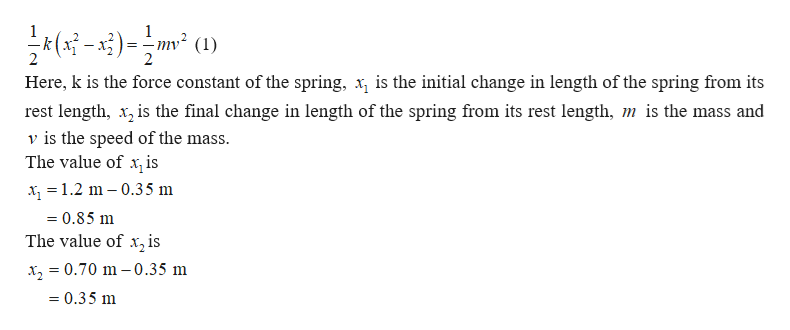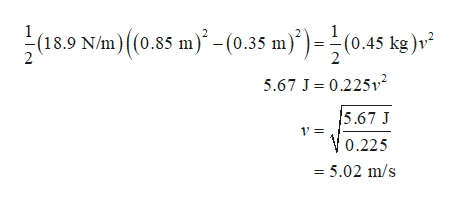# A spring has a force constant of 18.9 N/m and a rest length of 0.35 m.The spring is stretched to a length of 1.2 m and attached to a mass of 0.45 kg. When the spring is released, what is the speed of the mass when the spring has contracted to 0.70 m long?

Question
20 views
A spring has a force constant of 18.9 N/m and a rest length of 0.35 m.The spring is stretched to a length of 1.2 m and attached to a mass of 0.45 kg. When the spring is released, what is the speed of the mass when the spring has contracted to 0.70 m long?
check_circle

Step 1

The change in potential energy of the spring must be equal to the kinetic energy gained by the mass when it is released. This can be expressed ashelp_outlineImage TranscriptioncloseHere, k is the force constant of the spring, is the initial change in length of the spring from its rest length, x, is the final change in length of the spring from its rest length, m is the mass and v is the speed of the mass. The value of x is x =1.2 m 0.35 m 0.85 m The value of x, is x, = 0.70 m -0.35 m = 0.35 m fullscreen
Step 2

Substitute the values in equ...help_outlineImage Transcriptionclose18.9 N/m) (0.85 m-(0.35 m))=(0.45 kg)v 5.67 J 0.225v2 5.67 J 1= 0.225 = 5.02 m/s fullscreen

### Want to see the full answer?

See Solution

#### Want to see this answer and more?

Solutions are written by subject experts who are available 24/7. Questions are typically answered within 1 hour.*

See Solution
*Response times may vary by subject and question.
Tagged in

### Physics